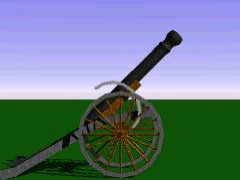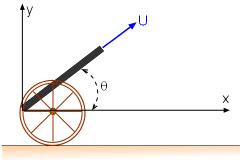Ch 10. Parametric Equations Multimedia Engineering Math ParametricEquations Tangentsand Areas Arc Length Surface Area
 Chapter 1. Limits 2. Derivatives I 3. Derivatives II 4. Mean Value 5. Curve Sketching 6. Integrals 7. Inverse Functions 8. Integration Tech. 9. Integrate App. 10. Parametric Eqs. 11. Polar Coord. 12. Series Appendix Basic Math Units Search eBooks Dynamics Fluids Math Mechanics Statics Thermodynamics Author(s): Hengzhong Wen Chean Chin Ngo Meirong Huang Kurt Gramoll ©Kurt GramollMATHEMATICS - CASE STUDY IntroductionProjectile Fired from a CannonProblem Diagram To prepare for a western movie set, Steve would like to find out the exact time and distance that a projectile will travel after it was fired from a cannon. It is known that the path of the projectile is given by the following parametric equations:      x = (Ucosθ)t      y = (Usinθ)t - 0.5gt2 In these equations, U is the initial velocity, θ is the angle above the horizontal, and g is the gravitational acceleration. Questions If the initial velocity of the projectile is 400 ft/s and the angle θ is 60o. Steve would like to find out how long and how far does it take to hit the ground. Plot the trajectory of the projectile. Approach Assume air resistance is negligible. The gravitational acceleration, g, is 32.2 ft/s2.